# Class 9 Maths Important questions for Circles

Given below are the Class 9 Maths Important questions for Circles
a) Concepts questions
b) Calculation problems
c) Multiple choice questions
e) Fill in the blank's
1) ABCD is a cyclic quadrilateral in which AC and BD are its diagonals. If < DBC= 550 and < BCD.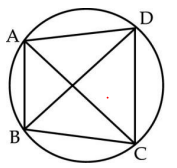2) In the figure O is the centre of the circle and < ABC = 450 . Show that OA is perpendicular to  OC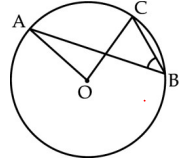3) Two concentric circles are with center O. ABCD are the points of intersection with a line. If AD = 12 cm and BC = 8 cm find the length of AB, CD, AC and BD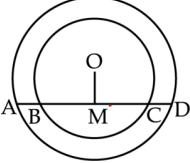4) ABCD is a cyclic quadrilateral whose diagonals intersect at a point E. If ∠ DBC = 70°, ∠ BAC = 30°, find ∠ BCD. Further, if AB = BC, find ∠ ECD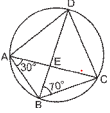5) Bisectors of angles A, B and C of a triangle ABC intersect its circumcircle at D, E and F respectively. Prove that the angles of the triangle DEF are 90° – (1 /2 )A, 90° – (1/ 2) B and 90° – (1 /2) C.
6) Two circles of radii 5 cm and 3 cm intersect at two points and the distance between their centres is 4 cm. Find the length of the common chord.

7) Two chords AB and CD of lengths 5 cm and 11 cm respectively of a circle are parallel to each other and are on opposite sides of its centre. If the
distance between AB and CD is 6 cm, find the radius of the circle
8) . AB and AC are two chords of circle of radius r such that AB = 2AC. If p and q are the distances of AB and AC from centre, prove that 4q2 = p2 + 3r2.
9) A quadrilateral PQRS is inscribed in a circle such that PQ is a diameter and <PSR = 130. Find <QPR
10) In below figure two chords AB and CD of a circle intersect at right angle. If <ABD =65, find the measure of <CAB.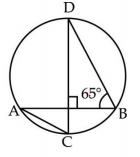Go back to Class 10 Main Page using below links

### Practice Question

Question 1 What is $1 - \sqrt {3}$ ?
A) Non terminating repeating
B) Non terminating non repeating
C) Terminating
D) None of the above
Question 2 The volume of the largest right circular cone that can be cut out from a cube of edge 4.2 cm is?
A) 19.4 cm3
B) 12 cm3
C) 78.6 cm3
D) 58.2 cm3
Question 3 The sum of the first three terms of an AP is 33. If the product of the first and the third term exceeds the second term by 29, the AP is ?
A) 2 ,21,11
B) 1,10,19
C) -1 ,8,17
D) 2 ,11,20